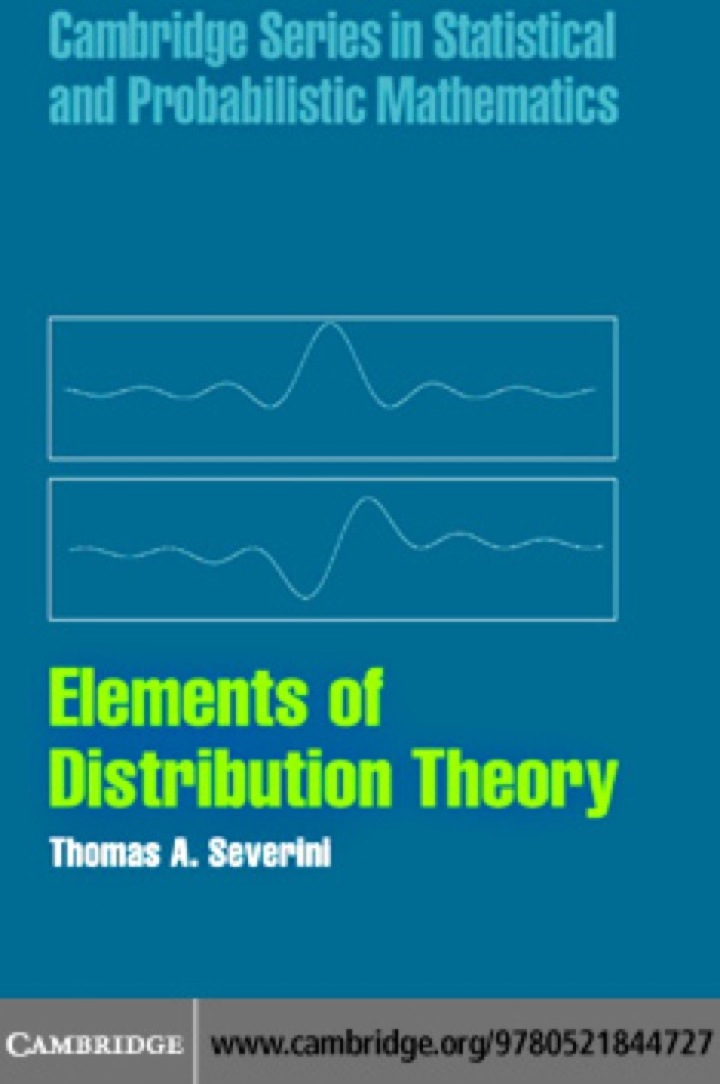# Elements of Distribution Theory

## Elements of Distribution Theory

By Thomas A. Severini

### Rent or Buy eTextbook

Expires on Apr 23rd, 2022
\$44

Publisher List Price: \$0.00

This detailed introduction to distribution theory uses no measure theory, making it suitable for students in statistics and econometrics as well as for researchers who use statistical methods. Good backgrounds in calculus and linear algebra are important and a course in elementary mathematical analysis is useful, but not required. An appendix gives a detailed summary of the mathematical definitions and results that are used in the book. Topics covered range from the basic distribution and density functions, expectation, conditioning, characteristic functions, cumulants, convergence in distribution and the central limit theorem to more advanced concepts such as exchangeability, models with a group structure, asymptotic approximations to integrals, orthogonal polynomials and saddlepoint approximations. The emphasis is on topics useful in understanding statistical methodology; thus, parametric statistical models and the distribution theory associated with the normal distribution are covered comprehensively.

Subject: Mathematics & Statistics -> Mathematics -> Probability1st edition
Publisher: Cambridge University Press 8/8/05
Imprint: Cambridge University Press
Language: English

ISBN 10: 0511343248
ISBN 13: 9780511343247
Print ISBN: 9780521844727

Live Chats